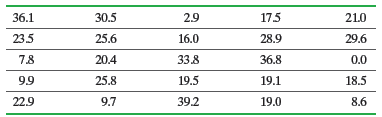×
Get Full Access to Statistics: Informed Decisions Using Data - 5 Edition - Chapter 7.3 - Problem 12
Get Full Access to Statistics: Informed Decisions Using Data - 5 Edition - Chapter 7.3 - Problem 12

×

# ?Hours of TV A random sample of college students aged 18–24 years was obtained, and the number of hours of television watched in a typical week was recISBN: 9780134133539 240

## Solution for problem 12 Chapter 7.3

Statistics: Informed Decisions Using Data | 5th Edition

• Textbook Solutions
• 2901 Step-by-step solutions solved by professors and subject experts
• Get 24/7 help from StudySoup virtual teaching assistantsStatistics: Informed Decisions Using Data | 5th Edition

4 5 1 421 Reviews
14
1
Problem 12

Hours of TV A random sample of college students aged 18–24 years was obtained, and the number of hours of television watched in a typical week was recorded.(a) Draw a normal probability plot to determine if the data could have come from a normal distribution.

(b) Determine the mean and standard deviation of the sample data.

(c) Using the sample mean and sample standard deviation obtained in part (b) as estimates for the population mean and population standard deviation, respectively, draw a graph of a normal model for the distribution of weekly hours of television watched.

(d) Using the normal model from part (c), find the probability that a college student aged 18–24 years, selected at random, watches between 20 and 35 hours of television each week.

(e) Using the normal model from part (c), determine the proportion of college students aged 18–24 years who watch more than 40 hours of television per week.

Step-by-Step Solution:

Step 1 of 5) Hours of TV A random sample of college students aged 18–24 years was obtained, and the number of hours of television watched in a typical week was recorded. (a) Draw a normal probability plot to determine if the data could have come from a normal distribution. (b) Determine the mean and standard deviation of the sample data. (c) Using the sample mean and sample standard deviation obtained in part (b) as estimates for the population mean and population standard deviation, respectively, draw a graph of a normal model for the distribution of weekly hours of television watched. (d) Using the normal model from part (c), find the probability that a college student aged 18–24 years, selected at random, watches between 20 and 35 hours of television each week. (e) Using the normal model from part (c), determine the proportion of college students aged 18–24 years who watch more than 40 hours of television per week. Next assign ranks to each observation (see Table 12) and add the ranks corresponding to model X (because this group corresponds to the median on the left in the hypothesis).

Step 2 of 5

Step 3 of 5

## Discover and learn what students are asking

Calculus: Early Transcendental Functions : Partial Derivatives
?In Exercises 7 - 38, find both first partial derivatives. $$z=y e^{y / x}$$

Unlock Textbook Solution